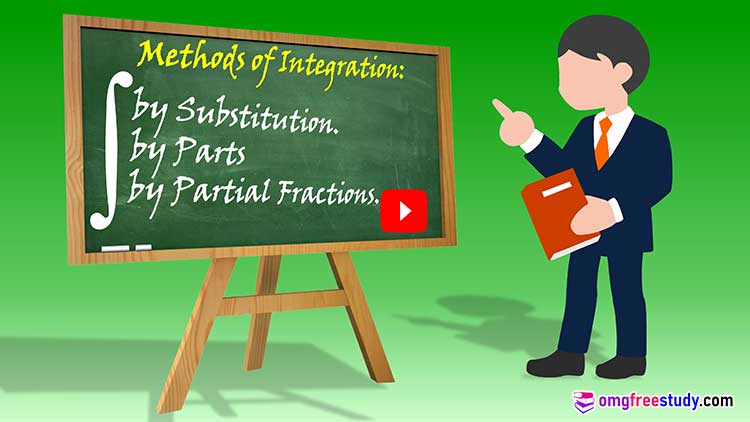# Integral Calculus | Methods of Integration

In integration by parts, we can solve the product of two functions are given to us then we apply the required formula. This method of solving is called LIATE rule. The integral of the two functions are taken, by considering the left hand term as first function and second term as the second function.## LIATE Rule

Suppose, we have question from exercise 6 question number 1 to integrate x ex, then we consider x as first function and ex as the second function.

So basically, the first function is chosen by using LIATE rule, in such a way that the derivative of the function could be easily integrated or integral.

Usually, the preference order of this rule is based on some functions such as Logarithm, Inverse, Algebraic, Trigonometric and Exponential as given below.

Used LIATE rule for selection of “u” and “v” function

Where,

L          =          Logarithmic Function

I           =          Inverse Trigonometric Function

A         =          Algebraic Function

T          =          Trigonometric Function

E          =          Exponential Function

Examples

Q.1: Find ∫ x cos x dx

Solution: Given, ∫ x cos x dx

The integral here is the product of two functions.

Therefore, we have to apply the formula of integral by parts called LIATE Rule.

So, x is an algebraic function and cos x is a trigonometric function. Therefor by LIATE rule, let x is equal to u as first function and cos x equal to v as second function.

u = x and v = cos x

Now, using the formula,

∫u . v dx = u ∫ v – ∫[∫v dx.  (du/dx)] dx

∫x cox x dx = x ∫ cos x – ∫ [∫ cos x dx. (dx/dx)] dx

∫x cox x dx = x sinx – ∫ [sin x . 1] dx

∫x cox x dx = x sinx – ∫ [sin x ] dx

∫x cox x dx = x sinx – (– cos x) + c

∫x cox x dx = x sinx + cos x + c

Where c is a constant.

## Methods of Integration:

• Integration by substitution.
• Integration by parts
• Integration by partial fractions.